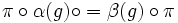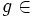# Using group actions and representations to solve the extensible automorphisms problem

(diff) ← Older revision | Latest revision (diff) | Newer revision → (diff)
This is a survey article related to:Extensible automorphisms problem
View other survey articles about Extensible automorphisms problem

An automorphism$\sigma$ of a group$G$ is termed extensible if, for any group$H$ containing$G$, there is an automorphism$\sigma'$ of$H$ whose restriction to$G$ equals$\sigma$.

The extensible automorphisms problem asks for a characterization of all the extensible automorphisms of any group. Clearly, any inner automorphism is extensible. The extensible automorphisms conjecture states that the only extensible automorphisms are the inner automorphisms.

Two main methods of attack for the problem are the use of group actions on sets and the use of linear representations, i.e., group actions on vector spaces. This article discusses a general common idea behind these methods, and the reasons behind the partial success as well as the limitations of such approaches.

## The main steps of the approach

### Step one: Reduce extensibility of the automorphism to being extensible to an inner automorphism with respect to that kind of action

Suppose$S$ is a structure with an automorphism group$A = \operatorname{Aut}(S)$. (The automorphisms are the automorphisms that preserve certain specified structural properties of$S$, these may be all permutations, linear maps, algebra automorphisms, self-homeomorphisms, isometries, or other things). A representation of$G$ on$S$ is a homomorphism$\alpha: G \to \operatorname{Aut}(S)$. Two representations$\alpha:G \to \operatorname{Aut}(S)$ and$\beta:G \to \operatorname{Aut}(T)$ are equivalent if there is an isomorphism$\pi:S \to T$ such that, for any$g \in G$:$\pi \circ \alpha(g) \circ = \beta(g) \circ \pi$.

In particular, if$S = T$, two representations of$G$ on$S$ are equivalent if there is an automorphism of$S$ such that conjugation by that automorphism in$\operatorname{Aut}(S)$ sends one homomorphism to the other.

The first step is to use the fact that an automorphism is extensible to deduce that for certain kinds of representations, it can be extended to an inner automorphism of the automorphism group. In other words, we would like to say that if$\sigma$ is an extensible automorphism of$G$, then for any representation$\alpha$ of$G$ on a structure$S$,$\alpha$ and$\alpha \circ \sigma$ are equivalent, i.e., there exists an element of$\operatorname{Aut}(S)$ that conjugates$\alpha(g)$ to$\alpha(\sigma(g))$ for all$g \in$.

This is tricky, and the way it is achieved is different in the linear representations and group actions case: